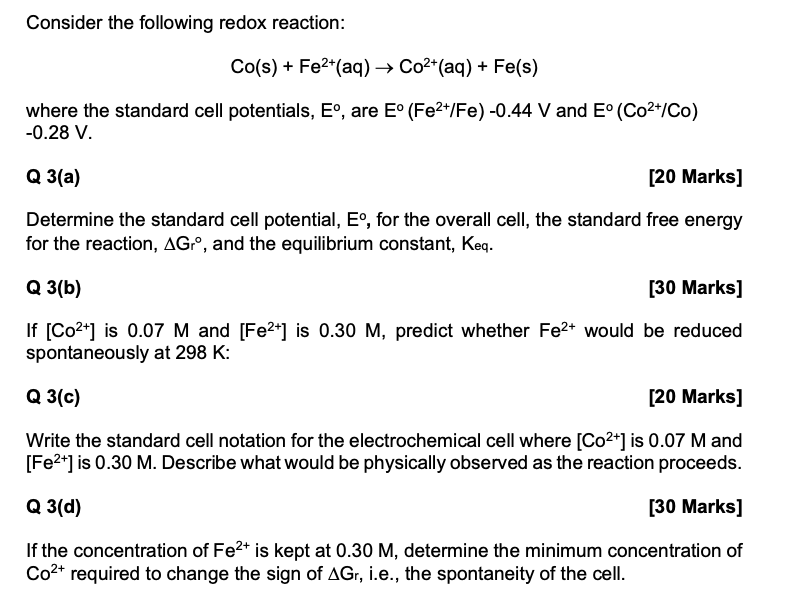### Create an Account

Home / Questions / Consider the following redox reaction: Co(s) + Fe2+(aq) → Co2+(aq) + Fe(s) where the stand...

# Consider the following redox reaction: Co(s) + Fe2+(aq) → Co2+(aq) + Fe(s) where the standard cell potentials, Eº, are E° (Fe2+/Fe) -0.44 V and Eº (Co2+/Co) -0.28 V.

Consider the following redox reaction: Co(s) + Fe2+(aq) → Co2+(aq) + Fe(s) where the standard cell potentials, Eº, are E° (Fe2+/Fe) -0.44 V and Eº (Co2+/Co) -0.28 V. Q 3(a) [20 Marks] Determine the standard cell potential, Eº, for the overall cell, the standard free energy for the reaction, AGr°, and the equilibrium constant, Keq. Q 3(b) [30 Marks] If [Co2+] is 0.07 M and [Fe2+] is 0.30 M, predict whether Fe2+ would be reduced spontaneously at 298 K: Q 3(c) [20 Marks] Write the standard cell notation for the electrochemical cell where [Co2+] is 0.07 M and [Fe2+] is 0.30 M. Describe what would be physically observed as the reaction proceeds. Q 3(d) [30 Marks] If the concentration of Fe2+ is kept at 0.30 M, determine the minimum concentration of Co2+ required to change the sign of AGr, i.e., the spontaneity of the cell.May 17 2021 View more View LessSubscribe To Get Solution